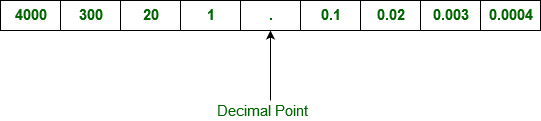GFG App
Open AppBrowser
Continue

# GRE Arithmetic | Decimals

The value of a digit can be determined by using its digit, position of digit in the number and base of the given number system.

Decimal number system has base 10 as it uses 10 digits from 0 to 9 and the successive positions to the left of the decimal point are as: 100, 101, 102, 103, … and so on. Successive positions to the right of the decimal point are as: 10-1, 10-2, 10-3, … and so on.

Example:
For example, the digits of the number 4321.1234 have following place values:4321.1234 can be written as,

```= (4 x 1000)+(3 x 100)+(2 x 10)+(1 x l)
+ (1 x 0.1)+(2 x 0.01)+(3 x 0.001)+(4 x 0.0001)

= (4 x 103)+(3 x 102)+(2 x 101)+(1 x 100)
+ (1 x 10-1)+(2 x 10-2)+(3 x 10-3)+(4 x 10-4)

= 4000 + 300 + 20 + 1 + 0.1 + 0.02 + 0.003 + 0.0004
= 4321.1234 ```

Since each place value is a power of 10, every decimal can be converted to an integer divided by a power of 10. We can convert a fractional decimal number to its equivalent fraction with integers in numerator and denominator.

Example-1:

`4.5 = 4 + 5/10 = 40/10 + 5/10 = 45/10`

Example-2:

`75.18 = 75 + 18/100 = 7500/100 + 18/100 = 7518/100`

Example-3:

`0.369 = 0 + 369/1000 = 369/1000`

We can also convert an Integer in numerator and denominator to its equivalent decimal fraction by dividing numerator by denominator. The result will either terminate or repeat without end. Repeating part of a decimal can be indicate using bar over repeating digits.

Example-4:

`3/4 = 0.75`

Example-5:

`134/20 = 6.7`

Example-6:

`30/28 = 1.0714285`

Note:

• Every Rational number can be expressed as a terminating or repeating decimal and converse is also true.
• Irrational numbers can not be expressed in numerator/denominatr form because these numbers neither have repeating fraction (i.e., √2 = 1.41421356237) nor has finite fraction (0.03033033303333…).
My Personal Notes arrow_drop_up